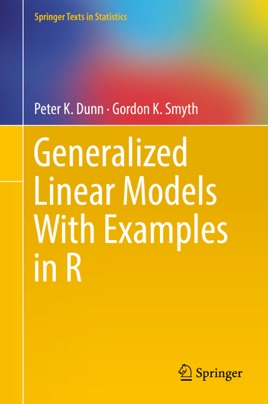• 87,99 €

## Description de l’éditeur

This textbook presents an introduction to multiple linear regression, providing real-world data sets and practice problems. A practical working knowledge of applied statistical practice is developed through the use of these data sets and numerous case studies. The authors include a set of practice problems both at the end of each chapter and at the end of the book. Each example in the text is cross-referenced with the relevant data set, so that readers can load the data and follow the analysis in their own R sessions. The balance between theory and practice is evident in the list of problems, which vary in difficulty and purpose.
This book is designed with teaching and learning in mind, featuring chapter introductions and summaries, exercises, short answers, and simple, clear examples. Focusing on the connections between generalized linear models (GLMs) and linear regression, the book also references advanced topics and tools that have not typically been included in introductions to GLMs to date, such as Tweedie family distributions with power variance functions, saddlepoint approximations, likelihood score tests, modified profile likelihood, and randomized quantile residuals. In addition, the authors introduce the new R code package, GLMsData, created specifically for this book. Generalized Linear Models with Examples in R balances theory with practice, making it ideal for both introductory and graduate-level students who have a basic knowledge of matrix algebra, calculus, and statistics.